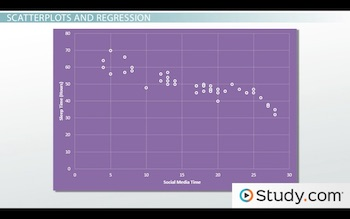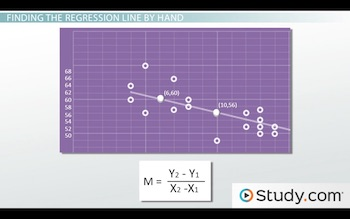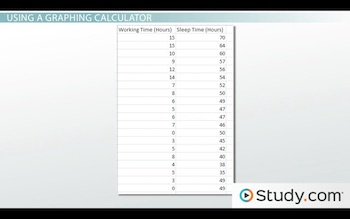# Simple Linear Regression: Definition, Formula & Examples

An error occurred trying to load this video.

Try refreshing the page, or contact customer support.

Coming up next: Problem Solving Using Linear Regression: Steps & Examples

### You're on a roll. Keep up the good work!

Replay
Your next lesson will play in 10 seconds
• 0:02 Simple Linear Regression
• 1:02 Scatterplots and Regression
• 2:36 Finding the Regression…
• 3:53 Using a Graphing Calculator
• 7:21 Practice Problems
• 8:47 Lesson Summary

Want to watch this again later?

Timeline
Autoplay
Autoplay
Speed

#### Recommended Lessons and Courses for You

Lesson Transcript
Instructor: Cathryn Jackson

Cat has taught a variety of subjects, including communications, mathematics, and technology. Cat has a master's degree in education and is currently working on her Ph.D.

Simple linear regression is a great way to make observations and interpret data. In this lesson, you will learn to find the regression line of a set of data using a ruler and a graphing calculator.

## Simple Linear Regression

Hannah is a scientist studying the time management and study skills of college students. She conducts an experiment at a local college with 50 students. She asks each student to track their time spent on social media, time spent studying, time spent sleeping and time spent working over the course of a semester. She also asks the students to record their final GPA for the semester.

In this lesson, you will be learning about the simple linear regression and how to find a regression line using a graphing calculator. A regression line is a straight line that attempts to predict the relationship between two points, also known as a trend line or line of best fit. Simple linear regression is a prediction when a variable (y) is dependent on a second variable (x) based on the regression equation of a given set of data. We'll see how Hannah uses simple linear regression to help interpret her data.

## Scatterplots and Regression

Once all 50 students turn in their data, Hannah creates a scatterplot, which is a graph of ordered pairs showing a relationship between two sets of data. For her first scatterplot, Hannah uses two variables: time spent on social networking and amount of sleep. To simplify her information, we are going to look at the average time per week each student spent sleeping and on social media. Take a look at the scatterplot:Since we are using two variables, we can call this bivariate data. Bivariate data is two sets of variables that can change and are compared to find relationships. Bivariate data is most often displayed visually using a scatterplot.

You may notice that this data has several points that create a sort of pattern. Many of the points increase in the x value as they decrease in the y value. This is a relationship between the two sets of data known as a correlation. A correlation is the relationship between two sets of variables used to describe or predict information.

A regression line is one way of predicting this information and finding a correlation in the data. There are two ways you can find the regression line of a set of data. The first way is to find the regression line by using a ruler, and the second way is to use a graphing calculator. Let's talk briefly about how to find a regression line by hand before we use a calculator.

## Finding the Regression Line by hand

To find a regression line by hand, follow these steps:

1. Draw a line that is closest to as many points as possible.
2. Choose two points, and calculate slope.
3. Write the equation of the line.

Let's use the scatterplot above to practice finding the regression line using a ruler.

First, use the ruler to find a place that is closest to as many points as possible. Sometimes you can find two points to use.

Second, pick two points you think would be on the regression line. You can use points that are on the line or you can make up new points. Use these points and plug them into the following equation:If you are unfamiliar with using this equation, check out our algebra lessons!

Third, now you have the slope of your line. You can create an equation based on this information. Find the y-intercept by extending the line all the way to the y-axis.

Use the slope-intercept equation to create the equation for your line like this:

• y = mx + b
y = -1x + 66

## Using a Graphing Calculator

Now that you know how to find a regression line by hand, let's talk about how to find a regression line using a calculator. Every calculator is a little bit different. However, you should be able to get by with just about any graphing calculator using these steps:

1. Set the calculator to Statistics mode.
2. Enter the data (1st Set on L1, 2nd Set on L2).
3. Adjust settings for a scatterplot, and then graph the points.
4. Set the calculator for regression line.

We will use the data that Hannah collected about the amount of sleep and the amount of work the students did during the semester:This data is a weekly average for each student. To save time, I'm only using 20 students, rather than the original 50. In this example, I am using a TI-83 graphing calculator.

First, set your calculator to statistics by pressing the Stat button. This will take you to a screen with the options of Edit, Calc and Tests. Select the Edit option by pressing enter. The L1 is the x-coordinates, and the L2 is for the y-coordinates. Enter each of the coordinates using the number pad and hitting 'Enter' when you are done entering each coordinate. After hitting the enter button, the calculator will take you to the next line for the second coordinate. You can also use the arrow buttons to move between L1 and L2.

Next, adjust the settings on your calculator to display a scatterplot. To do this, hit the 2nd button then hit Statplot. You should see this screen:Hit Enter to go into the next screen, which looks like this:To unlock this lesson you must be a Study.com Member.

### Register to view this lesson

Are you a student or a teacher?

### Unlock Your Education

#### See for yourself why 30 million people use Study.com

##### Become a Study.com member and start learning now.
Back
What teachers are saying about Study.com

### Earning College Credit

Did you know… We have over 160 college courses that prepare you to earn credit by exam that is accepted by over 1,500 colleges and universities. You can test out of the first two years of college and save thousands off your degree. Anyone can earn credit-by-exam regardless of age or education level.